# Prove: A x B = ∅ iff.  A = ∅ or B = ∅

Prove: A x B = ∅ iff.  A = ∅ or B = ∅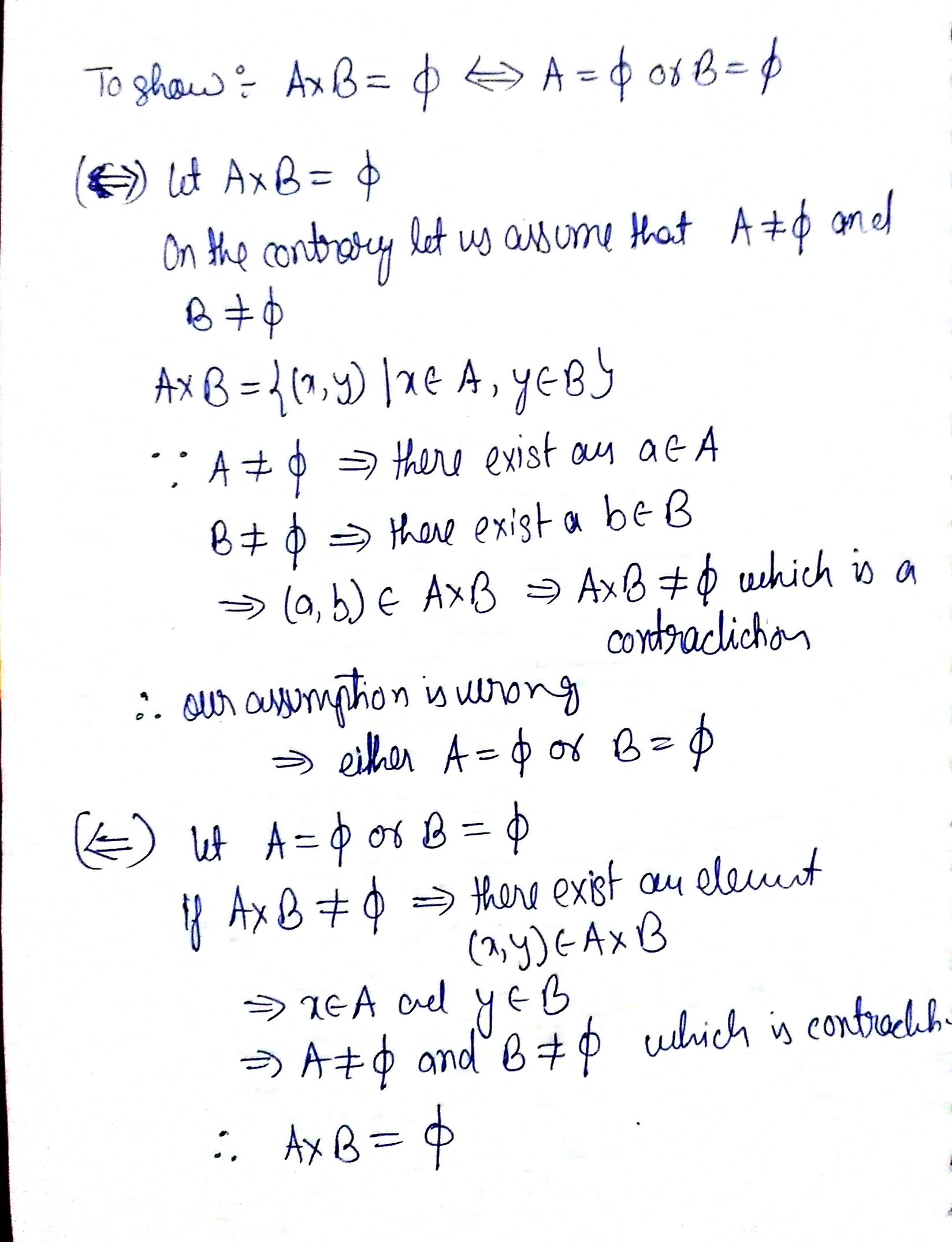##### Add Answer of: Prove: A x B = ∅ iff.  A = ∅ or B = ∅
Similar Homework Help Questions
• ### Prove: there exists x and y for c=ax+by iff gcd(a,b)/c

Prove: there exists x and y for c=ax+by iff gcd(a,b)/c.

• ### 10. Prove that a connected surface M is orientable iff there exists a nonvanishing differential 2-form on M 10. Prove t...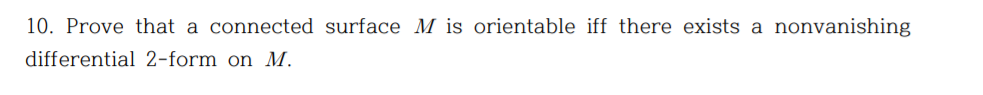10. Prove that a connected surface M is orientable iff there exists a nonvanishing differential 2-form on M 10. Prove that a connected surface M is orientable iff there exists a nonvanishing differential 2-form on M. 10. Prove that a connected surface M is orientable iff there exists a nonvanishing differential 2-form on M.

• ### prove AC>AB iff (beta)>(gamma).

Let triangle ABC have angles (alpha), (beta), (gamma), where (alpha) = angle BAC, etc. The side opposite (alpha) is the segement BC, etc.Prove that AC>AB iff (beta)>(gamma).Hint: give a proof by contradiction that uses =>

• ### 4. (5 points) Let A and B ben x n matrices. Prove that if A and...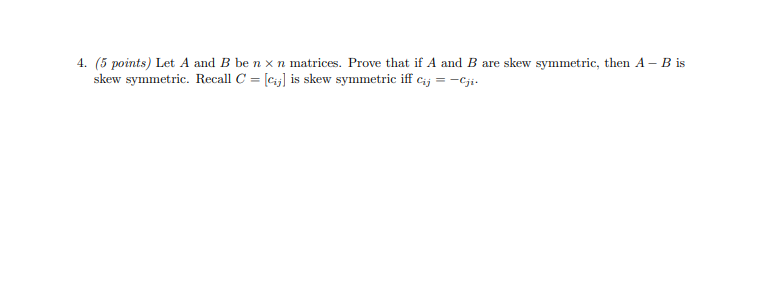4. (5 points) Let A and B ben x n matrices. Prove that if A and B are skew symmetric, then A - B is skew symmetric. Recall C = [cj] is skew symmetric iff Cij =-Cji.

• ### 1. Let V be a vector space with bases B and C. Suppose that T:V V is a linear map with matrix representations Ms(T)A and Me(T) B. Prove the following (a) T is one-to-one iff A is one-to-one. (b...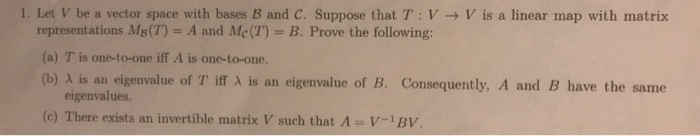1. Let V be a vector space with bases B and C. Suppose that T:V V is a linear map with matrix representations Ms(T)A and Me(T) B. Prove the following (a) T is one-to-one iff A is one-to-one. (b) λ is an eigenvalue of T iff λ is an eigenvalue of B. Consequently, A and B have the same eigenvalues (c) There exists an invertible matrix V such that A-V-BV 1. Let V be a vector space with bases B...

• ### In Z (integers) define the relation xRy iff x and y have the same tens digit

In Z (integers) define the relation xRy iff x and y have the same tens digit. Prove or disprove R as a equivalence relation.

• ### 9. Prove that (a - b) x (a+ b) = 2(a x b) X 9. Prove that (a - b) x (a+ b) = 2(a x b) X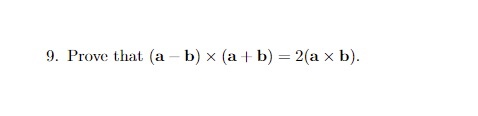9. Prove that (a - b) x (a+ b) = 2(a x b) X 9. Prove that (a - b) x (a+ b) = 2(a x b) X

• ### 2. Let f : A ! B. DeÖne a relation R on A by xRy i§ f (x) = f (y). a. Prove that R is an equivalence relation on A. b. Let Ex = fy 2 A : xRyg be the equivalence class of x 2 A. DeÖne E = fEx : x 2 Ag...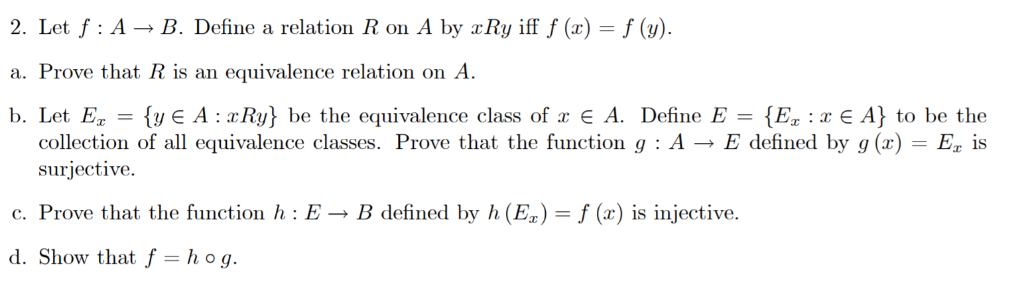2. Let f : A ! B. DeÖne a relation R on A by xRy i§ f (x) = f (y). a. Prove that R is an equivalence relation on A. b. Let Ex = fy 2 A : xRyg be the equivalence class of x 2 A. DeÖne E = fEx : x 2 Ag to be the collection of all equivalence classes. Prove that the function g : A ! E deÖned by g (x) = Ex is...

• ### 3. (i) Prove that the set of all linear combinations of a and b are precisely...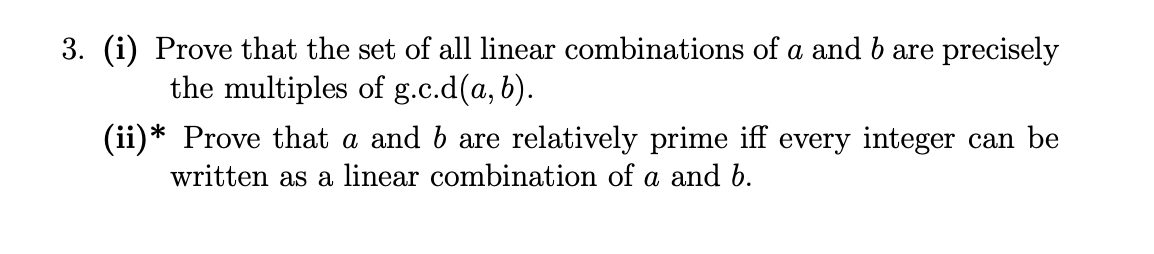3. (i) Prove that the set of all linear combinations of a and b are precisely the multiples of g.c.d(a,b). (ii)* Prove that a and b are relatively prime iff every integer can be written as a linear combination of a and b.

• ### Prove that if x ∉ B and A ⊆ B, then x ∉ A

Prove that if x ∉ B and A ⊆ B, then x ∉ A.

Free Homework App